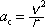centripetal acceleration = speed-squared divided by radius

A point particle that is moving in a circle has an acceleration a-sub-c, directed toward the center of the circle, that is equal in magnitude to the square-of-the-speed of the particle divided by the radius r of the circle the particle is moving on.

Note: A particle that is moving in a circle has centripetal acceleration whether or not the speed of the particle is changing. If the speed of the particle is changing, the particle has tangential acceleration too and the total acceleration is the vector sum of the centripetal acceleration and the tangential acceleration.

Pitfall Avoidance Note: For a particle to have an acceleration directed toward the center of the circle, there must be a force, acting on the particle, which is also directed toward the center of the circle. While such a force is referred to as a centripetal force, it should be realized that the centripetal force is not some new kind of force that arises as a result of circular motion, rather, it is an ordinary force (such as the tension in some string, the gravitational force of the sun on a planet, a frictional force exerted on car tires by the surface of a circular race track, etc.) that is the force responsible for causing the object to move in a circle rather than the straight line path that the particle would move on if there was no force. Never put a force labeled Fc for centripetal force on a free body diagram. Label the centripetal force T for tension, or FG for gravitational, or N for normal, or fK for frictional, etc., in accord with the agent of the force rather than in accord with the name of the kind of acceleration that the force causes.